# 吴恩达《Machine Learning》精炼笔记 2：梯度下降与正规方程

• 多维特征
• 多变量梯度下降
• 梯度下降法实践
• 正规方程
• 多维特征Multiple Features

## 多维特征Multiple Features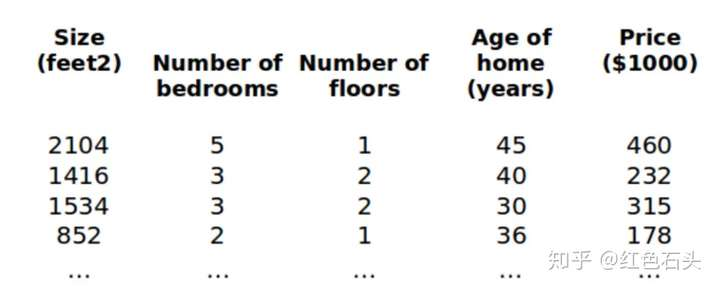n：代表的是特征的数量

x(i)：代表第i个训练实例，是特征矩阵中的第i行，是一个向量vector

xij：表示的是第i个训练实例的第j个特征；i表示行，j表示列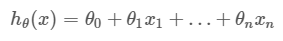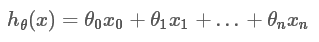## 多变量梯度下降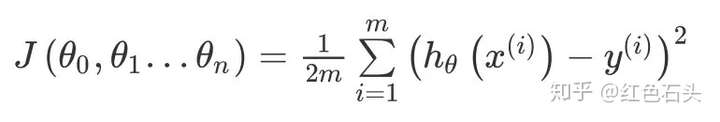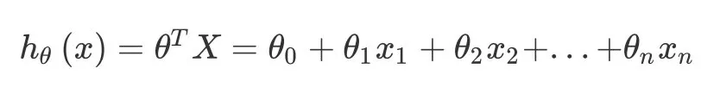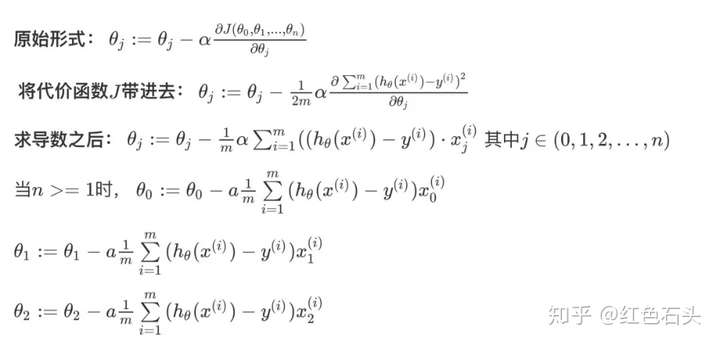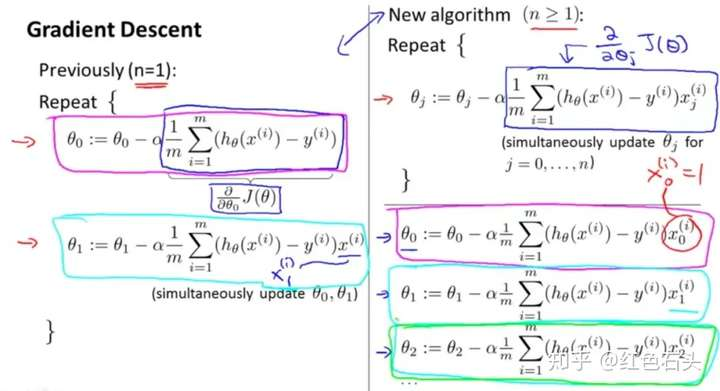Python代码

import numpy as np

def computeCost(X,y,theta):
inner = np.power(((X * theta.T) - y), 2)  # 求解每个平方项
return np.sum(inner) / (2 / len(X))   # 求和再除以2*len(X)


## 梯度下降法实践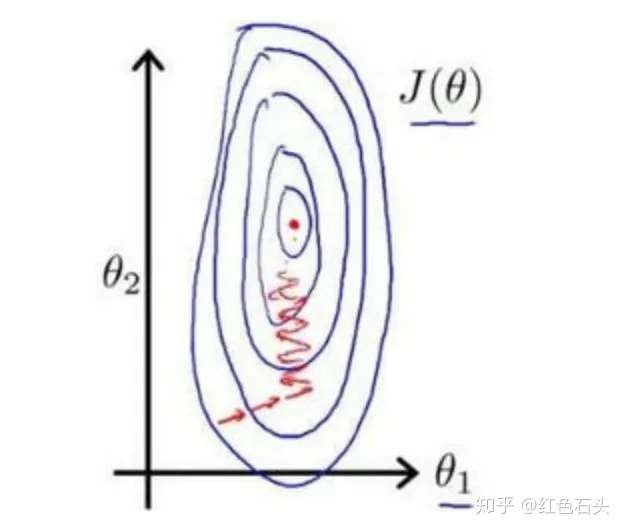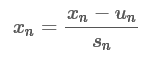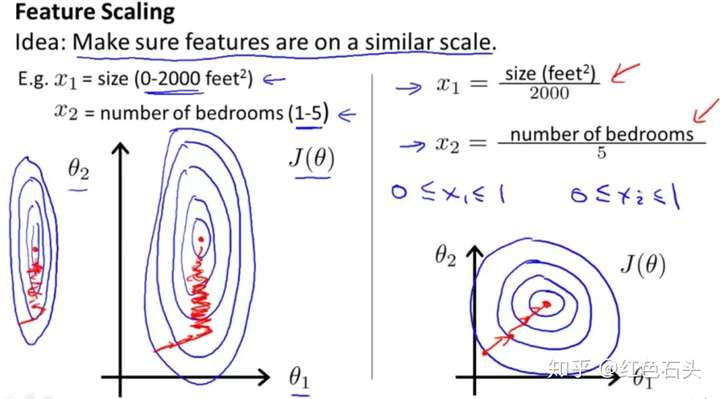## 均值归一化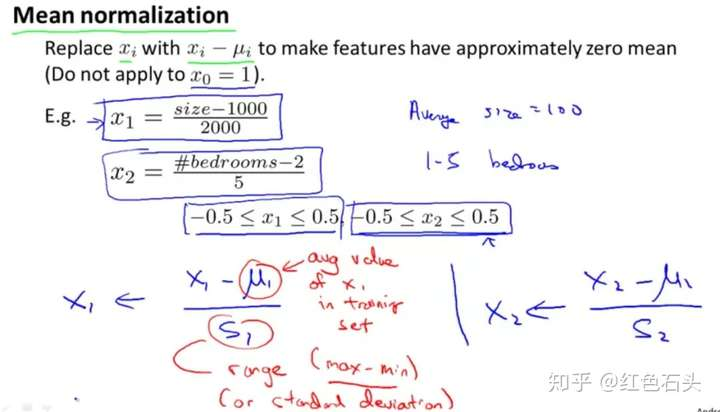## 学习率问题

• 如果学习率过小，则达到收敛所需的迭代次数会非常高，收敛速度非常慢
• 如果学习率过大，每次迭代可能不会减小代价函数，可能会越过局部最小值导致无法收敛

## 特征和多项式回归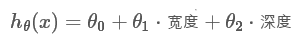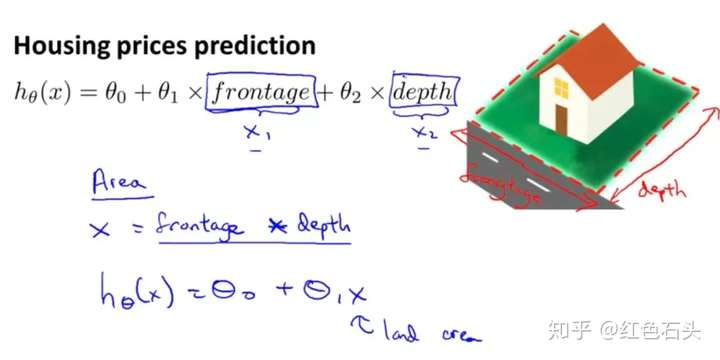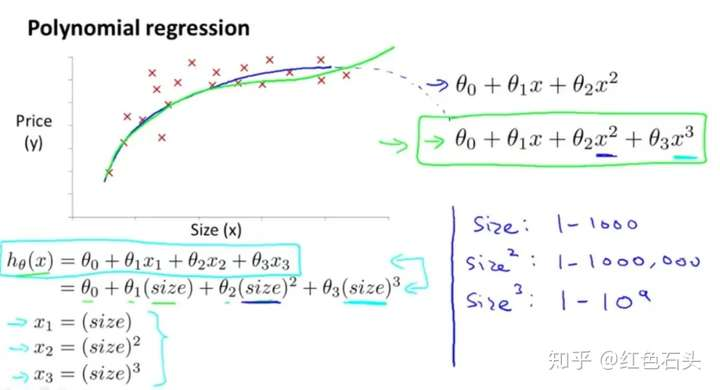## 正规方程 Normal Equation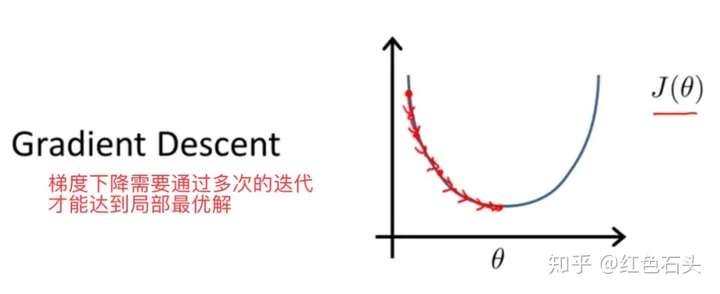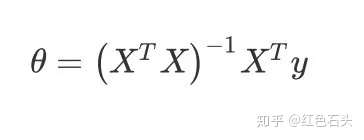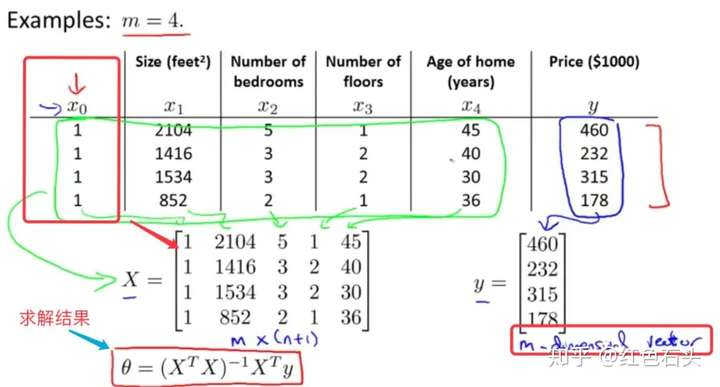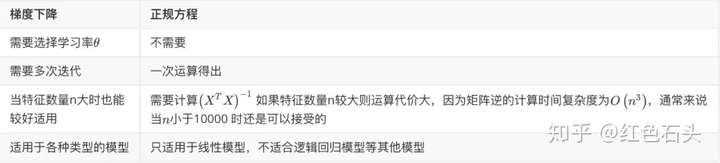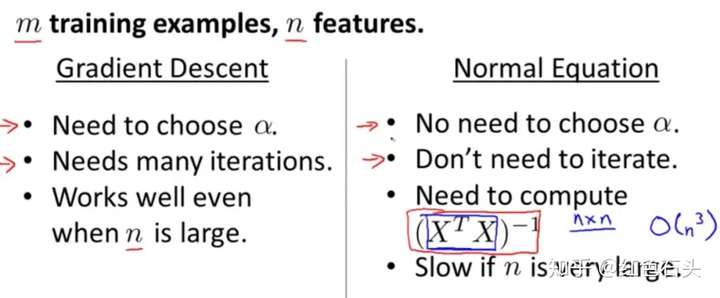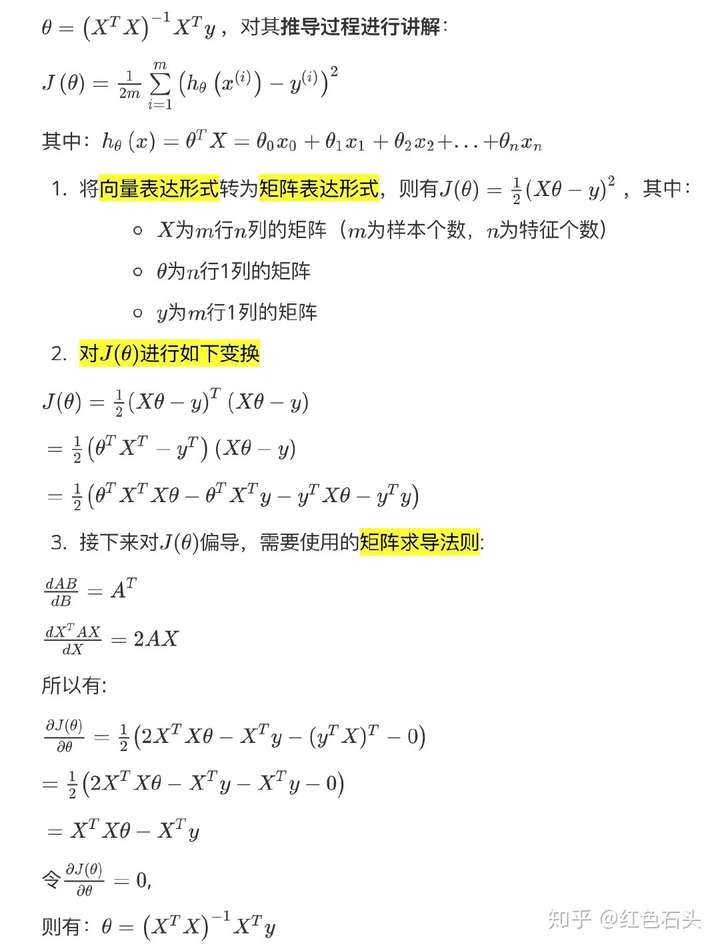import numpy as np

def normalEquation(X, y):
theta = np.linalg.inv(X.T@X)@X.T@Y   # X.T@X等价于X.T.dot(X)  @等价于.dot
return theta### 觉得文章有用就打赏一下文章作者

#### 支付宝扫一扫打赏#### 微信扫一扫打赏